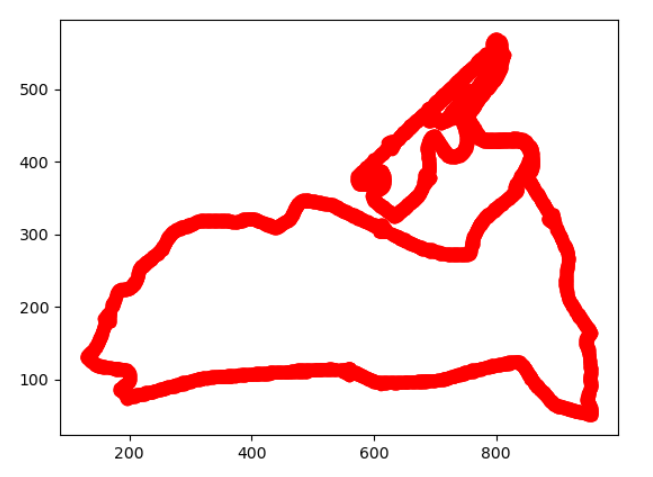# 编程实战（4）——python识别图像中的坐标点并保存坐标数据

### 文章目录

• 编程实战（4）——python识别图像中的坐标点并保存坐标数据
• 综述
• 代码思路
• 库的安装
• 图片预处理
• 图像细化
• 图像二极化
• 提取数据
• 结果展示和保存
• matplotlib重绘
• 写入excel
• 全部源码
• ### 综述

最近因为美赛的需求，需要在提取一些赛道的路线图和地形图中的准确数据，因此对这方面做了一些了解。在研究的过程中，我发现网上的很多相关的帖子并不是很靠谱，不是报错就是没有说清楚一些函数的功能，所以我打算写一篇比较详细的文章。

本文主要讲述利用python接口的opencv来完成图像识别和信息提取并重新绘制、保存为excel数据的详细过程与思路，适合opencv方面的小白观看（需要一定的numpy和matplotlib基础）。如有一些疏漏，请大佬们指出~

### 代码思路

我会跟着我代码的思路逐一讲解每一步的思路和函数的一些解释；

总体思路如下：

• 第一：图片预处理，让图像二极化；
• 第二：提取图片数据
• 第三步：数据整理与保存
• #### 库的安装

这里一共用了三个库

``````import cv2
import numpy as np
import matplotlib.pyplot as plt
``````

numpy和matplotlib的安装都比较常规，但是cv2的安装不是常规的pip install cv2，是opencv-python，国内镜像下载地址：

``````pip3 install -i https://mirrors.aliyun.com/pypi/simple/ opencv-python
``````

#### 图片预处理

``````img = cv2.imread('你的图片路径')
hsv = cv2.cvtColor(img, cv2.COLOR_BGR2HSV)
``````

首先打开图片，然后对图片做一个hsv灰度图的处理，因为我们常规的图片像素点都是rbg空间模式的，我们需要先转化为hsv颜色空间模式（没见过这个图像格式的可以百度一下），以便后面的图像二值化处理；上面是我用到的图像，这是东京奥运会公路自行车比赛的路线图，我们要做的就是提取路线像素并坐标化；

##### 图像细化

如果说要处理的图像的线条很粗，那就会影响后面的识别过程，需要先进行图像细化；如果原本图的线条就很细（比如我的），那就可以跳过这一步。

图像细化我是直接参考一个博客的代码的，这里做一个引用：图像细化，骨架提取

写的相当棒，大家可以参考一下。

##### 图像二极化

这里我用到了一个cv库中的inRange函数，这个函数的功能是对读入的图像文件（即函数第一个参数）做一个二值化处理。

总的来说就是我们需要规定两个阈值lowerb和upperb，大于upperb和小于lowerb的图像像素点均会被转化为0（即黑色），在这个范围内的点被转化为255（白色）；

``````low_hsv = np.array([0, 0, 221])
high_hsv = np.array([180, 30, 255])
``````

我们再来详细的讲一下这两个阈值，如果了解hsv颜色空间的话，很容易可以发现代码的前两行就是hsv颜色空间的两个值，numpy.array函数里面的参数刚好就是色调、饱和度和明度值，因为inRange函数的需要，我们要转化成numpy格式的数组。我这里需要提取所有黑色的坐标，所以设的是黑色与白色的阈值，大家可以根据自己的需求调整颜色阈值。

阈值参考：HSV基本颜色分量范围

#### 提取数据

``````print(len(mask))
``````

输出：

``````654
1024
[255 255 255 ... 255 255 255]
[255 255 255 ... 255 255 255]
...
[255 255 255 ... 255 255 255]
[255 255 255 ... 255 255 255]
[255 255 255 ... 255 255 255]
``````

可以看到图像是1024*654的，还有每一行的像素点都是一个numpy数组（numpy数组打印出来元素之间是没有用逗号间隔的），之前24位的hsv图，变成了8位的灰度图（每一项只有一个数据，而不是三个数组成的array）；

方法也很简单，直接遍历每一个像素，找到值为0的像素点，存取列号和行号即可；

``````list_y = []
list_x = []

xmax = []
list_x.append(j)
``````

#### 结果展示和保存

##### matplotlib重绘

检验一下我们获取的图像数据，注意这里需要用散点图模式绘图，不然会有不太好的后果。。。

``````plt.plot(list_x, list_y, 'o', color='r')
plt.show()
``````

结果如下：可以发现提取效果还是不错的~~

##### 写入excel

这一部分的说明直接放到注释里面了

``````import xlwt

wb = xlwt.Workbook()

ws.write(0, 0, "x") # 写入数据，3个参数分别为行号，列号，和内容
ws.write(0, 1, "y")

i = 1 #指针，每写一个数据，向下移动写指针一行
for x in list_x:
ws.write(i, 0, x)
i += 1

j = 1
for y in list_y:
ws.write(j, 1, y)
j += 1

wb.save('1111.xls')
``````

可以看到数据已经保存进excel里面了这一次提取其实还有一个小问题，就是相同的横坐标下还是有多个对应该横坐标的点，如果需要做函数分析之类的操作，我们可以直接用excel作按值分组然后去每组的特定值即可，最大值，平均值均可，看个人需求。

### 全部源码

``````import cv2
import numpy as np
import matplotlib.pyplot as plt
import xlwt

hsv = cv2.cvtColor(img, cv2.COLOR_BGR2HSV)
low_hsv = np.array([0, 0, 221])
high_hsv = np.array([180, 30, 255])

list_y = []
list_x = []

xmax = []
list_x.append(j)

plt.plot(list_x, list_y, 'o', color='r')
plt.show()

wb = xlwt.Workbook()

ws.write(0, 0, "x")
ws.write(0, 1, "y")
i = 1
for x in list_x:
ws.write(i, 0, x)
i += 1

j = 1
for y in list_y:
ws.write(j, 1, y)
j += 1

wb.save('1111.xls')
``````

来源：伊滴小朋友